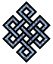#fail2ban bad ip database: ip 178.128.65.102

### | ip database | live view | stats | report | help | api key:

 ip: 178.128.65.102 hostname: 178.128.65.102 country:[GR] Greece first reported: 20.11.2018 11:56.53 GMT+0200 last reported: 14.12.2018 18:37.14 GMT+0200 time period: 24d 06h 40m 21s total reports: 10 reported by: 3 host(s) filter(s): ssh (7) ssh (3) tor exit node no badips.com db Lookup## port scan of '178.128.65.102':

[-hide]
```# Nmap 6.40 scan initiated Tue Nov 20 11:57:01 2018 as: /usr/bin/nmap -sU -sS -O 178.128.65.102
Nmap scan report for 178.128.65.102
Host is up (0.15s latency).
Not shown: 1000 open|filtered ports, 995 filtered ports
PORT     STATE  SERVICE
20/tcp   closed ftp-data
21/tcp   open   ftp
22/tcp   closed ssh
3000/tcp closed ppp
8080/tcp closed http-proxy
No exact OS matches for host (If you know what OS is running on it, see http://nmap.org/submit/ ).
TCP/IP fingerprint:
OS:SCAN(V=6.40%E=4%D=11/20%OT=21%CT=20%CU=%PV=N%G=Y%TM=5BF3E8B0%P=x86_64-pc
OS:-linux-gnu)SEQ(SP=105%GCD=1%ISR=108%TI=Z%CI=I%TS=8)SEQ(SP=105%GCD=1%ISR=
OS:108%TI=Z%TS=8)OPS(O1=M5B4ST11NW7%O2=M5B4ST11NW7%O3=M5B4NNT11NW7%O4=M5B4S
OS:T11NW7%O5=M5B4ST11NW7%O6=M5B4ST11)WIN(W1=7120%W2=7120%W3=7120%W4=7120%W5
OS:=7120%W6=7120)ECN(R=Y%DF=Y%TG=40%W=7210%O=M5B4NNSNW7%CC=Y%Q=)T1(R=Y%DF=Y
OS:%TG=40%S=O%A=S+%F=AS%RD=0%Q=)T2(R=N)T3(R=N)T4(R=Y%DF=Y%TG=40%W=0%S=A%A=Z
OS:%F=R%O=%RD=0%Q=)T5(R=Y%DF=Y%TG=40%W=0%S=Z%A=S+%F=AR%O=%RD=0%Q=)T6(R=Y%DF
OS:=Y%TG=40%W=0%S=A%A=Z%F=R%O=%RD=0%Q=)T7(R=N)U1(R=N)IE(R=Y%DFI=N%TG=40%CD=
OS:S)

OS detection performed. Please report any incorrect results at http://nmap.org/submit/ .
# Nmap done at Tue Nov 20 11:57:52 2018 -- 1 IP address (1 host up) scanned in 51.29 seconds
```
```Σ = 48 | Δt = 0.0037381649017334s
```